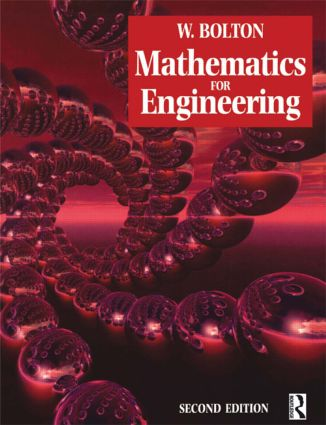Mathematics for Engineering

2nd Edition

Routledge

350 pages

Purchasing Options:\$ = USD
Paperback: 9780750649315
pub: 2000-05-15
SAVE ~\$9.79
\$48.95
\$39.16
x
Hardback: 9781138174597
pub: 2016-02-01
SAVE ~\$37.00
\$185.00
\$148.00
x
eBook (VitalSource) : 9780080939292
pub: 2012-08-06
from \$24.48

FREE Standard Shipping!

Description

Mathematics for Engineering has been carefully designed to provide a maths course for a wide ability range, and does not go beyond the requirements of Advanced GNVQ. It is an ideal text for any pre-degree engineering course where students require revision of the basics and plenty of practice work.

Bill Bolton introduces the key concepts through examples set firmly in engineering contexts, which students will find relevant and motivating.

The second edition has been carefully matched to the Curriculum 2000 Advanced GNVQ units:
Applied Mathematics in Engineering (compulsory unit 5)
Further Mathematics for Engineering (Edexcel option unit 13)
Further Applied Mathematics for Engineering (AQA / City & Guilds option unit 25)

A new introductory section on number and mensuration has been added, as well as a new section on series and some further material on applications of differentiation and definite integration.

Bill Bolton is a leading author of college texts in engineering and other technical subjects. As well as being a lecturer for many years, he has also been Head of Research, Development and Monitoring at BTEC and acted as a consultant for the Further Education Unit.

Preface; NUMBER AND MENSURATION: Units and powers; Mensuration; ALGEBRA: Algebraic equations; Series; Linear equations; Simultaneous equations; Quadratic equations; Logarithmic and exponential equations; Electrical circuit algebra; Mechanics algebra. TRIGONOMETRY: Trigonometric ratios; Trigonometric identities; Trigonometric formulas; Vector trigonometry. GRAPHS: Cartesian graphs; Linear graphs; Non-linear graphs; Logarithmic graphs; Electrical circuit graphs; Mechanics graphs. CALCULUS: Introduction to differentiation; Derivatives; Introduction to integration; Electrical circuit calculus; Mechanics calculus; Answers; Index